# Cantor's diagonal argument facts for kids

Kids Encyclopedia Facts

Cantor's diagonal argument is a mathematical method to prove that two infinite sets have the same cardinality. Cantor published articles on it in 1877, 1891 and 1899. His first proof of the diagonal argument was published in 1890 in the journal of the German Mathematical Society (Deutsche Mathematiker-Vereinigung). According to Cantor, two sets have the same cardinality, if it is possible to associate an element from the second set to each element of the first set, and to associate an element of the first set to each element of the second set. This statement works well for sets with a finite number of elements. It is less intuitive for sets with an infinite number of elements.

## Cantor's first diagonal argument

The example below uses two of the simplest infinite sets, that of natural numbers, and that of positive fractions. The idea is to show that both of these sets,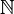$\mathbb{N}$ and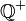$\mathbb{Q^+}$ have the same cardinality.

First, the fractions are aligned in an array, as follows: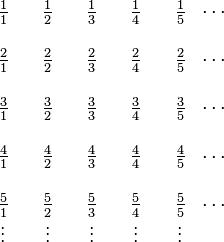$\begin{array}{cccccccccc} \tfrac 11 & & \tfrac 12 & & \tfrac 13 & & \tfrac 14 & & \tfrac 15 & \cdots \\ & & & & & & & & & \\ \tfrac 21 & & \tfrac 22 & & \tfrac 23 & & \tfrac 24 & & \tfrac 25 & \cdots \\ & & & & & & & & & \\ \tfrac 31 & & \tfrac 32 & & \tfrac 33 & & \tfrac 34 & & \tfrac 35 & \cdots \\ & & & & & & & & & \\ \tfrac 41 & & \tfrac 42 & & \tfrac 43 & & \tfrac 44 & & \tfrac 45 & \cdots \\ & & & & & & & & & \\ \tfrac 51 & & \tfrac 52 & & \tfrac 53 & & \tfrac 54 & & \tfrac 55 & \cdots \\ \vdots & & \vdots & & \vdots & & \vdots & & \vdots & \\ \end{array}$

Next, the numbers are counted, as shown. Fractions which can be simplified are left out: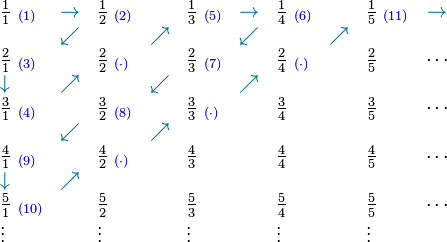$\begin{array}{lclclclclc} \tfrac 11\ _{\color{Blue} (1)} & {\color{MidnightBlue}\rightarrow} & \tfrac 12\ _{\color{Blue} (2)} & & \tfrac 13\ _{\color{Blue} (5)} & {\color{MidnightBlue}\rightarrow} & \tfrac 14\ _{\color{Blue} (6)} & & \tfrac 15\ _{\color{Blue} (11)} & {\color{MidnightBlue}\rightarrow} \\ & {\color{MidnightBlue}\swarrow} & & {\color{MidnightBlue}\nearrow} & & {\color{MidnightBlue}\swarrow} & & {\color{MidnightBlue}\nearrow} & & \\ \tfrac 21\ _{\color{Blue} (3)} & & \tfrac 22\ _{\color{Blue} (\cdot)} & & \tfrac 23\ _{\color{Blue} (7)} & & \tfrac 24\ _{\color{Blue} (\cdot)} & & \tfrac 25 & \cdots \\ {\color{MidnightBlue}\downarrow} & {\color{MidnightBlue}\nearrow} & & {\color{MidnightBlue}\swarrow} & & {\color{MidnightBlue}\nearrow} & & & & \\ \tfrac 31\ _{\color{Blue} (4)} & & \tfrac 32\ _{\color{Blue} (8)} & & \tfrac 33\ _{\color{Blue} (\cdot)} & & \tfrac 34 & & \tfrac 35 & \cdots \\ & {\color{MidnightBlue}\swarrow} & & {\color{MidnightBlue}\nearrow} & & & & & & \\ \tfrac 41\ _{\color{Blue} (9)} & & \tfrac 42\ _{\color{Blue} (\cdot)} & & \tfrac 43 & & \tfrac 44 & & \tfrac 45 & \cdots \\ {\color{MidnightBlue}\downarrow} & {\color{MidnightBlue}\nearrow} & & & & & & & & \\ \tfrac 51\ _{\color{Blue} (10)} & & \tfrac 52 & & \tfrac 53 & & \tfrac 54 & & \tfrac 55 & \cdots \\ \vdots & & \vdots & & \vdots & & \vdots & & \vdots & \\ \end{array}$

That way, the fractions are counted: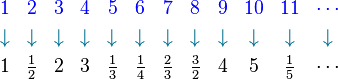$\begin{array}{cccccccccccccccc} {\color{Blue} 1} & {\color{Blue} 2} & {\color{Blue} 3} & {\color{Blue} 4} & {\color{Blue} 5} & {\color{Blue} 6} & {\color{Blue} 7} & {\color{Blue} 8} & {\color{Blue} 9} & {\color{Blue} 10} & {\color{Blue} 11} & {\color{Blue} \cdots} \\[3pt] {\color{MidnightBlue}\downarrow} & {\color{MidnightBlue}\downarrow} & {\color{MidnightBlue}\downarrow} & {\color{MidnightBlue}\downarrow} & {\color{MidnightBlue}\downarrow} & {\color{MidnightBlue}\downarrow} & {\color{MidnightBlue}\downarrow} & {\color{MidnightBlue}\downarrow} & {\color{MidnightBlue}\downarrow} & {\color{MidnightBlue}\downarrow} & {\color{MidnightBlue}\downarrow} & {\color{MidnightBlue}\downarrow} \\[3pt] 1 & \tfrac 12 & 2 & 3 & \tfrac 13 & \tfrac 14 & \tfrac 23 & \tfrac 32 & 4 & 5 & \tfrac 15 & \cdots \\ \end{array}$

By omitting fractions that can still be simplified, there is a bijection which associates each element of the natural numbers, to one element of the fractions:

To show that all natural numbers and the fractions have the same cardinality, the element 0 needs to be added; after each fraction its negative is added;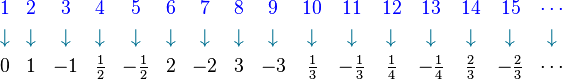$\begin{array}{cccccccccccccccc} {\color{Blue} 1} & {\color{Blue} 2} & {\color{Blue} 3} & {\color{Blue} 4} & {\color{Blue} 5} & {\color{Blue} 6} & {\color{Blue} 7} & {\color{Blue} 8} & {\color{Blue} 9} & {\color{Blue} 10} & {\color{Blue} 11} & {\color{Blue} 12} & {\color{Blue} 13} & {\color{Blue} 14} & {\color{Blue} 15} & {\color{Blue} \cdots} \\[3pt] {\color{MidnightBlue}\downarrow} & {\color{MidnightBlue}\downarrow} & {\color{MidnightBlue}\downarrow} & {\color{MidnightBlue}\downarrow} & {\color{MidnightBlue}\downarrow} & {\color{MidnightBlue}\downarrow} & {\color{MidnightBlue}\downarrow} & {\color{MidnightBlue}\downarrow} & {\color{MidnightBlue}\downarrow} & {\color{MidnightBlue}\downarrow} & {\color{MidnightBlue}\downarrow} & {\color{MidnightBlue}\downarrow} & {\color{MidnightBlue}\downarrow} & {\color{MidnightBlue}\downarrow} & {\color{MidnightBlue}\downarrow} & {\color{MidnightBlue}\downarrow} \\[3pt] 0 & 1 & -1 & \tfrac 12 & -\tfrac 12 & 2 & -2 & 3 & -3 & \tfrac 13 & -\tfrac 13 & \tfrac 14 & -\tfrac 14 & \tfrac 23 & -\tfrac 23 & \cdots \\ \end{array}$

That way, there is a complete bijection, which associates a fraction to each natural number. In other words: both sets have the same cardinality. Today, sets that have the same number of elements than the set of natural numbers are called countable. Sets which have fewer elements than the natural numbers are called at most countable. With that definition, the set of rational numbers / fractions is countable.

Infinite sets often have properties which go against intuition: David Hilbert showed this in an experiment which is called Hilbert's paradox of the Grand Hotel.

## Real numbers

The set of real numbers does not have the same cardinality than those of the natural numbers; there are more real numbers than natural numbers. The idea outlined above influenced his proof. In his 1891 article, Cantor considered the set T of all infinite sequences of binary digits (i.e. each digit is zero or one).

He begins with a constructive proof of the following theorem:

If s1, s2, … , sn, … is any enumeration of elements from T, then there is always an element s of T which corresponds to no sn in the enumeration.

To prove this, given an enumeration of elements from T, like e.g.

 s1 = (0, 0, 0, 0, 0, 0, 0, ...) s2 = (1, 1, 1, 1, 1, 1, 1, ...) s3 = (0, 1, 0, 1, 0, 1, 0, ...) s4 = (1, 0, 1, 0, 1, 0, 1, ...) s5 = (1, 1, 0, 1, 0, 1, 1, ...) s6 = (0, 0, 1, 1, 0, 1, 1, ...) s7 = (1, 0, 0, 0, 1, 0, 0, ...) ...

The sequence s is constructed by choosing the 1st digit as complementary to the 1st digit of s1 (swapping 0s for 1s and vice versa), the 2nd digit as complementary to the 2nd digit of s2, the 3rd digit as complementary to the 3rd digit of s3, and generally for every n, the nth digit as complementary to the nth digit of sn. In the example, this yields:

 s1 = (0, 0, 0, 0, 0, 0, 0, ...) s2 = (1, 1, 1, 1, 1, 1, 1, ...) s3 = (0, 1, 0, 1, 0, 1, 0, ...) s4 = (1, 0, 1, 0, 1, 0, 1, ...) s5 = (1, 1, 0, 1, 0, 1, 1, ...) s6 = (0, 0, 1, 1, 0, 1, 1, ...) s7 = (1, 0, 0, 0, 1, 0, 0, ...) ... s = (1, 0, 1, 1, 1, 0, 1, ...)

By construction, s differs from each sn, since their nth digits differ (highlighted in the example). Hence, s cannot occur in the enumeration.

Based on this theorem, Cantor then uses a proof by contradiction to show that:

The set T is uncountable.

He assumes for contradiction that T was countable. In that case, all its elements could be written as an enumeration s1, s2, … , sn, … . Applying the previous theorem to this enumeration would produce a sequence s not belonging to the enumeration. However, s was an element of T and should therefore be in the enumeration. This contradicts the original assumption, so T must be uncountable.Cantor's diagonal argument Facts for Kids. Kiddle Encyclopedia.